Next: Electricity Up: Vectors Previous: Surface Integrals

## Volume Integrals

A volume integral takes the form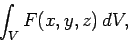(50)

where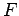is a three-dimensional mathematical function,some volume in space, and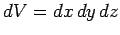an element of this volume. The volume element is sometimes written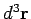.

As an example of a volume integral, let us evaluate the centre of gravity of a solid hemisphere of radius(centered on the origin). The height of the centre of gravity is given by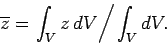(51)

The bottom integral is simply the volume of the hemisphere, which is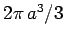. The top integral is most easily evaluated in spherical polar coordinates (,,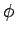), for which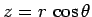and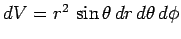. Thus,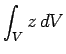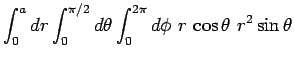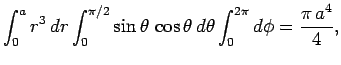(52)

giving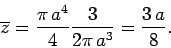(53)Next: Electricity Up: Vectors Previous: Surface Integrals
Richard Fitzpatrick 2007-07-14